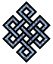#fail2ban bad ip database: ip 27.96.91.36

### | ip database | live view | stats | report | help | api key:

 ip: 27.96.91.36 hostname: 27.96.91.36 country: [??] UNKNOWN first reported: 16.11.2018 01:20.28 GMT+0200 last reported: 27.11.2018 07:15.22 GMT+0200 time period: 11d 05h 54m 54s total reports: 10 reported by: 2 host(s) filter(s): ssh (10) tor exit node no badips.com db Lookup## port scan of '27.96.91.36':

[-hide]
```# Nmap 6.40 scan initiated Fri Nov 16 01:21:01 2018 as: /usr/bin/nmap -sU -sS -O 27.96.91.36
Nmap scan report for 27.96.91.36
Host is up (0.13s latency).
Not shown: 1004 closed ports, 989 open|filtered ports
PORT     STATE SERVICE
22/tcp   open  ssh
25/tcp   open  smtp
80/tcp   open  http
443/tcp  open  https
3000/tcp open  ppp
3306/tcp open  mysql
8080/tcp open  http-proxy
No exact OS matches for host (If you know what OS is running on it, see http://nmap.org/submit/ ).
TCP/IP fingerprint:
OS:SCAN(V=6.40%E=4%D=11/16%OT=22%CT=1%CU=1066%PV=N%G=Y%TM=5BEE0DD4%P=x86_64
OS:-pc-linux-gnu)SEQ(SP=106%GCD=1%ISR=109%TI=Z%CI=I%TS=8)OPS(O1=M550ST11NW7
OS:%O2=M550ST11NW7%O3=M550NNT11NW7%O4=M550ST11NW7%O5=M550ST11NW7%O6=M550ST1
OS:1)WIN(W1=7120%W2=7120%W3=7120%W4=7120%W5=7120%W6=7120)ECN(R=Y%DF=Y%TG=40
OS:%W=7210%O=M550NNSNW7%CC=Y%Q=)T1(R=Y%DF=Y%TG=40%S=O%A=S+%F=AS%RD=0%Q=)T2(
OS:R=N)T3(R=N)T4(R=Y%DF=Y%TG=40%W=0%S=A%A=Z%F=R%O=%RD=0%Q=)T5(R=Y%DF=Y%TG=4
OS:0%W=0%S=Z%A=S+%F=AR%O=%RD=0%Q=)T6(R=Y%DF=Y%TG=40%W=0%S=A%A=Z%F=R%O=%RD=0
OS:%Q=)T7(R=N)U1(R=N)IE(R=N)

OS detection performed. Please report any incorrect results at http://nmap.org/submit/ .
# Nmap done at Fri Nov 16 01:22:44 2018 -- 1 IP address (1 host up) scanned in 103.19 seconds
```
```Σ = 32 | Δt = 0.0075719356536865s
```